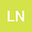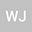A note on Liouville theorem for steady Q-tensor system of liquid crystal
••• Lai Ning-An,
• Wu Jiayan
Lai Ning-An
Lishui University

Corresponding Author:ninganlai@lsu.edu.cn

Author ProfileWu Jiayan
Zhejiang University
Author Profile## Abstract

We study the Liouville theorem for steady Q-tensor system of liquid crystal in $\R^3$. Assuming that $u\in L^{\frac 92, \infty}(\R^3)\cap \dot{H}(\R^3)$ and $Q\in H^2(\R^3)$, we show that the steady system admits only trivial solution $u=0, Q=0$.